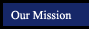Prentice Hall Algebra 1 California Edition
Chapter 11
 Section Title Tutorials from this book More examples (in pop-up window) 11.1 Real Numbers 11.2 Radical Expressions 11.3 Simplifing Radical Expressions 11.4 Multiplying Radicals Expressions 11.5 Dividing and Simplifing 11.6 Addition and Subtraction 11.7 The Pythagorean Theorem 11.8 Using the Pythagorean Theorem 11.9 Equations with Radicals
 Suggested Assignments Section Topic Pages Exercises 11.1 Real Numbers 482 11.2 Radical Expressions 487 11.3 Simplifing Radical Expressions 491 11.4 Multiplying Radicals Expressions 495 11.5 Dividing and Simplifing 498 11.6 Addition and Subtraction 504 11.7 The Pythagorean Theorem 509 11.8 Using the Pythagorean Theorem 514 11.9 Equations with Radicals 519 Preparing for Standardized Tests 523 Chapter 11 Wrap Up 525 Chapter 11 Assessment 528 Chapter 11 Cumlative Review 529

Online Scientific Calculator from Calculator.com

Graphing Calculator by CoolMath.com

Math Glossary (more sophisticated than it looks!)